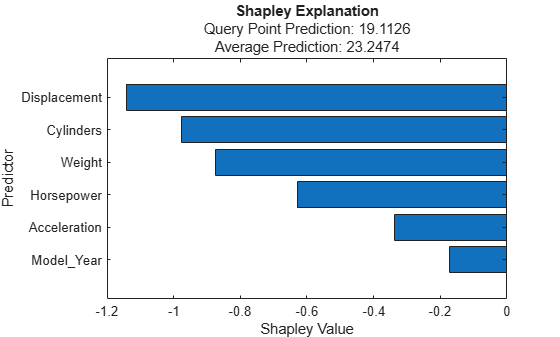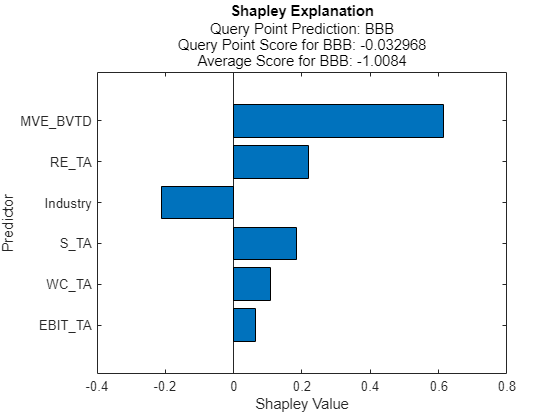fit

Compute Shapley values for query point

Description

example

newExplainer = fit(explainer,queryPoint) computes the Shapley values for the specified query point (queryPoint) and stores the computed Shapley values in the ShapleyValues property of newExplainer. The shapley object explainer contains a machine learning model and the options for computing Shapley values.

fit uses the Shapley value computation options that you specify when you create explainer. You can change the options using the name-value arguments of the fit function. The function returns a shapley object newExplainer that contains the newly computed Shapley values.

example

newExplainer = fit(explainer,queryPoint,Name,Value) specifies additional options using one or more name-value arguments. For example, specify 'UseParallel',true to compute Shapley values in parallel.

Examples

collapse all

Train a regression model and create a shapley object. When you create a shapley object, if you do not specify a query point, then the software does not compute Shapley values. Use the object function fit to compute the Shapley values for the specified query point. Then create a bar graph of the Shapley values by using the object function plot.

Load the carbig data set, which contains measurements of cars made in the 1970s and early 1980s.

Create a table containing the predictor variables Acceleration, Cylinders, and so on, as well as the response variable MPG.

tbl = table(Acceleration,Cylinders,Displacement,Horsepower,Model_Year,Weight,MPG);

Removing missing values in a training set can help reduce memory consumption and speed up training for the fitrkernel function. Remove missing values in tbl.

tbl = rmmissing(tbl);

Train a blackbox model of MPG by using the fitrkernel function

rng('default') % For reproducibility
mdl = fitrkernel(tbl,'MPG','CategoricalPredictors',[2 5]);

Create a shapley object. Specify the data set tbl, because mdl does not contain training data.

explainer = shapley(mdl,tbl)
explainer =
shapley with properties:

BlackboxModel: [1x1 RegressionKernel]
QueryPoint: []
BlackboxFitted: []
ShapleyValues: []
NumSubsets: 64
X: [392x7 table]
CategoricalPredictors: [2 5]
Method: 'interventional-kernel'
Intercept: 22.6202

explainer stores the training data tbl in the X property.

Compute the Shapley values of all predictor variables for the first observation in tbl.

queryPoint = tbl(1,:)
queryPoint=1×7 table
Acceleration    Cylinders    Displacement    Horsepower    Model_Year    Weight    MPG
____________    _________    ____________    __________    __________    ______    ___

12             8            307            130            70         3504     18

explainer = fit(explainer,queryPoint);

For a regression model, shapley computes Shapley values using the predicted response, and stores them in the ShapleyValues property. Display the values in the ShapleyValues property.

explainer.ShapleyValues
ans=6×2 table
Predictor       ShapleyValue
______________    ____________

"Acceleration"       -0.1561
"Cylinders"         -0.18306
"Displacement"      -0.34203
"Horsepower"        -0.27291
"Model_Year"         -0.2926
"Weight"            -0.32402

Plot the Shapley values for the query point by using the plot function.

plot(explainer)The horizontal bar graph shows the Shapley values for all variables, sorted by their absolute values. Each Shapley value explains the deviation of the prediction for the query point from the average, due to the corresponding variable.

Train a classification model and create a shapley object. Then compute the Shapley values for multiple query points.

Load the CreditRating_Historical data set. The data set contains customer IDs and their financial ratios, industry labels, and credit ratings.

Train a blackbox model of credit ratings by using the fitcecoc function. Use the variables from the second through seventh columns in tbl as the predictor variables.

blackbox = fitcecoc(tbl,'Rating', ...
'PredictorNames',tbl.Properties.VariableNames(2:7), ...
'CategoricalPredictors','Industry');

Create a shapley object with the blackbox model. For faster computation, subsample 25% of the observations from tbl with stratification and use the samples to compute the Shapley values. Specify to use the extension to the kernelSHAP algorithm.

rng('default') % For reproducibility
c = cvpartition(tbl.Rating,'Holdout',0.25);
tbl_s = tbl(test(c),:);
explainer = shapley(blackbox,tbl_s,'Method','conditional-kernel');

Find two query points whose true rating values are AAA and B, respectively.

queryPoint(1,:) = tbl_s(find(strcmp(tbl_s.Rating,'AAA'),1),:);
queryPoint(2,:) = tbl_s(find(strcmp(tbl_s.Rating,'B'),1),:)
queryPoint=2×8 table
ID      WC_TA     RE_TA     EBIT_TA    MVE_BVTD    S_TA     Industry    Rating
_____    ______    ______    _______    ________    _____    ________    _______

58258     0.511     0.869     0.106      8.538      0.732       2        {'AAA'}
82367    -0.078    -0.042     0.011      0.262      0.167       7        {'B'  }

Compute and plot the Shapley values for the first query point.

explainer1 = fit(explainer,queryPoint(1,:));
plot(explainer1)Compute and plot the Shapley values for the second query point.

explainer2 = fit(explainer,queryPoint(2,:));
plot(explainer2)The true rating for the second query point is B, but the predicted rating is BB. The plot shows the Shapley values for the predicted rating.

explainer1 and explainer2 include the Shapley values for the first query point and second query point, respectively.

Input Arguments

collapse all

Object explaining the blackbox model, specified as a shapley object.

Query point at which fit explains a prediction, specified as a row vector of numeric values or a single-row table.

• For a row vector of numeric values:

• The variables that makes up the columns of queryPoint must have the same order as the predictor data X in explainer.

• If the predictor data explainer.X is a table, then queryPoint can be a numeric vector if the table contains all numeric variables.

• For a single-row table:

• If the predictor data explainer.X is a table, then all predictor variables in queryPoint must have the same variable names and data types as those in explainer.X. However, the column order of queryPoint does not need to correspond to the column order of explainer.X.

• If the predictor data explainer.X is a numeric matrix, then the predictor names in explainer.BlackboxModel.PredictorNames and the corresponding predictor variable names in queryPoint must be the same. To specify predictor names during training, use the 'PredictorNames' name-value argument. All predictor variables in queryPoint must be numeric vectors.

• queryPoint can contain additional variables (response variables, observation weights, and so on), but fit ignores them.

• fit does not support multicolumn variables or cell arrays other than cell arrays of character vectors.

If queryPoint contains NaNs for continuous predictors and 'Method' is 'conditional-kernel', then the Shapley values (ShapleyValues) in the returned object are NaNs. Otherwise, fit handles NaN values in the same way as explainer.BlackboxModel (the predict object function of explainer.BlackboxModel or a function handle specified by blackbox).

Example: explainer.X(1,:) specifies the query point as the first observation of the predictor data X in explainer.

Data Types: single | double | table

Name-Value Arguments

Specify optional comma-separated pairs of Name,Value arguments. Name is the argument name and Value is the corresponding value. Name must appear inside quotes. You can specify several name and value pair arguments in any order as Name1,Value1,...,NameN,ValueN.

Example: fit(explainer,q,'Method','conditional-kernel','UseParallel',true) computes the Shapley values for the query point q using the extension to the kernelSHAP algorithm, and executes the computation in parallel.

Maximum number of predictor subsets to use for Shapley value computation, specified as a positive integer.

For details on how fit chooses the subsets to use, see Complexity of Computing Shapley Values.

Example: 'MaxNumSubsets',100

Data Types: single | double

Shapley value computation algorithm, specified as 'interventional-kernel' or 'conditional-kernel'.

• 'interventional-kernel'fit uses the kernelSHAP algorithm  with an interventional value function.

• 'conditional-kernel'fit uses the extension to the kernelSHAP algorithm  with a conditional value function.

For details about these algorithms, see Shapley Value Computation Algorithms.

Example: 'Method','conditional-kernel'

Data Types: char | string

Flag to run in parallel, specified as true or false. If you specify 'UseParallel',true, the fit function executes for-loop iterations in parallel by using parfor. This option requires Parallel Computing Toolbox™.

Example: 'UseParallel',true

Data Types: logical

Output Arguments

collapse all

Object explaining the blackbox model, returned as a shapley object. The ShapleyValues property of the object contains the computed Shapley values.

To overwrite the input argument explainer, assign the output of fit to explainer:

explainer = fit(explainer,queryPoint);

collapse all

Shapley Values

In game theory, the Shapley value of a player is the average marginal contribution of the player in a cooperative game. In the context of machine learning prediction, the Shapley value of a feature for a query point explains the contribution of the feature to a prediction (response for regression or score of each class for classification) at the specified query point.

The Shapley value of a feature for a query point is the contribution of the feature to the deviation from the average prediction. For a query point, the sum of the Shapley values for all features corresponds to the total deviation of the prediction from the average. That is, the sum of the average prediction and the Shapley values for all features corresponds to the prediction for the query point.

For more details, see Shapley Values for Machine Learning Model.

 Lundberg, Scott M., and S. Lee. "A Unified Approach to Interpreting Model Predictions." Advances in Neural Information Processing Systems 30 (2017): 4765–774.

 Aas, Kjersti, Martin. Jullum, and Anders Løland. "Explaining Individual Predictions When Features Are Dependent: More Accurate Approximations to Shapley Values." arXiv:1903.10464 (2019).